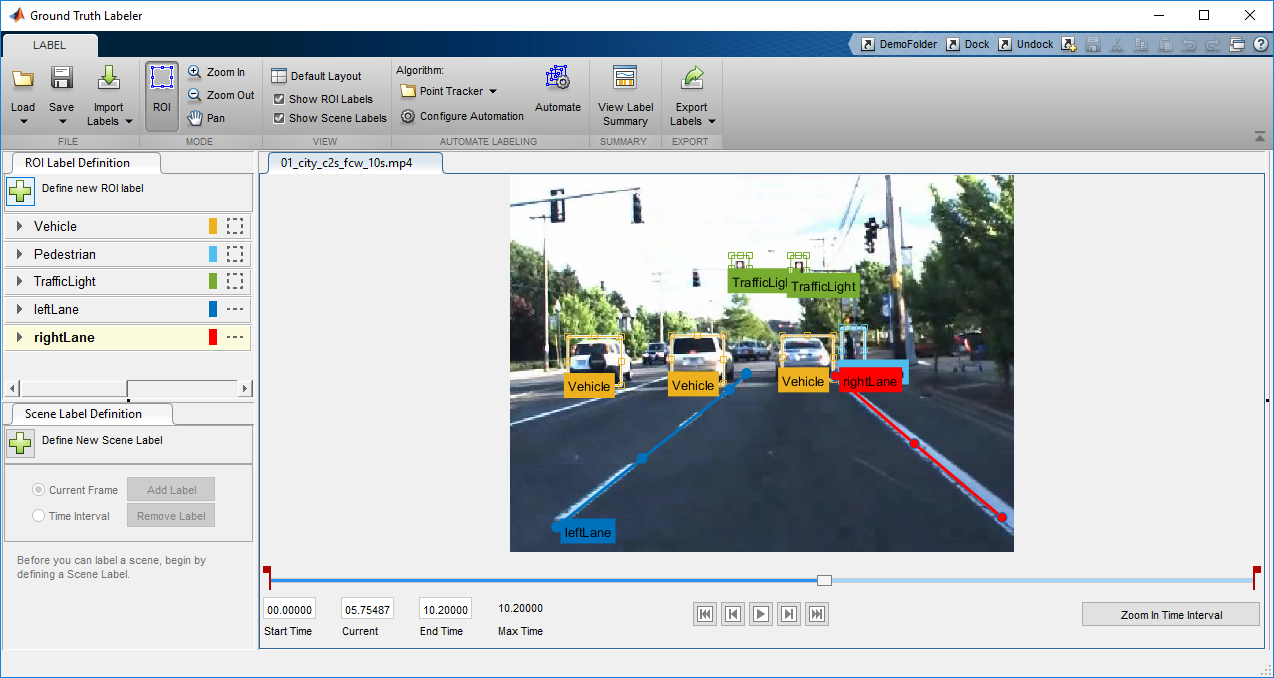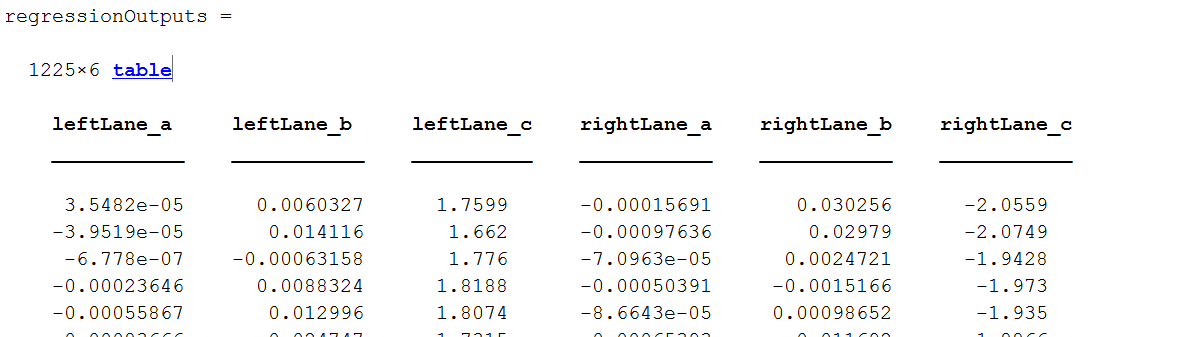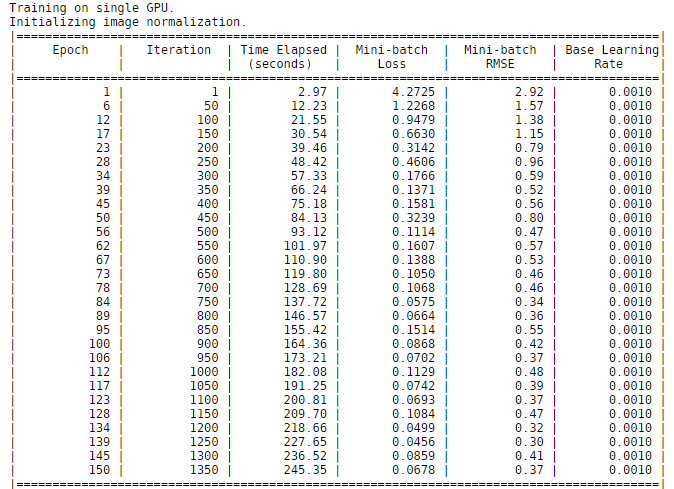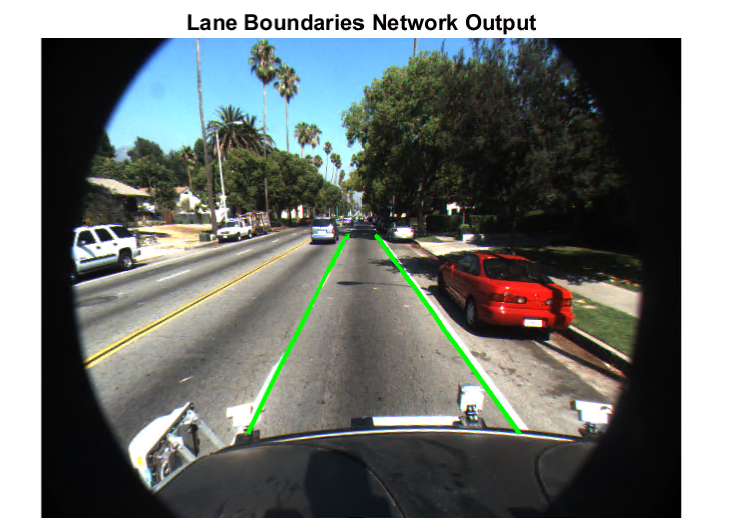# Deep Learning for Automated Driving (Part 2) – Lane Detection

This is the second post in the series on using deep learning for automated driving. In the first post I covered object detection (specifically vehicle detection). In this post I will go over how deep learning is used to find lane boundaries.

## Lane Detection

Lane detection is the identification of the location and curvature of lane boundaries of visible lanes on a roadway. This is useful to help a vehicle center it's driving path and safely navigate lane changes. Unlike the previous post where the algorithm had to predict the class of the vehicle (classification) as well as its location (bounding box), in this case I need the algorithm to output a set of numbers that represent the coefficients of parabolas that represent the right and left lane boundaries. To solve this, I will construct a CNN that performs regression to output the coefficients. Similar to my previous post, the first step in the process is to label a set of training data with the ground truth representing the right and left lane boundaries. As in the previous section,  I recommend using the Ground Truth Labeler app in MATLAB Automated Driving System Toolbox. Notice how I’ve labeled the lane boundaries using poly-lines in the figure below in addition to other objects that are labeled using rectangular bounding boxes.Labeled objects and lane boundaries.

To get a little insight on the ground truth for lane boundaries, the table shows the table used to store the coefficients. Notice each column represents one of the coefficients of the parabola.Coefficients of parabolas representing lane boundaries.

You’ll notice that there are just 1225 training samples for this task, which is usually not sufficient to train a deep network. The reason this actually works for us is I used transfer learning, by starting with a pre-existing network that was trained on a massive set of images and adapting it for our specific task of finding lane boundaries. The network I’ll use as a starting point is AlexNet, trained to recognize 1000 different categories of images. You can load a pre-trained AlexNet model into MATLAB with a single line of code. Note that MATLAB allows you to load other models like GoogLeNet, VGG-16 and VGG-19, or import models from the Caffe ModelZoo.
originalConvNet = alexnet
Once I have the network loaded into MATLAB I need to modify its structure slightly to change it from a classification network into a regression network. Notice in the code below that, I have 6 outputs corresponding to the three coefficients for the parabola representing each lane boundary(left and right).
% Extract layers from the original network
layers = originalConvNet.Layers

% Net surgery
% Replace the last few fully connected layers with suitable size layers
layers(20:25) = [];
outputLayers = [ ...
fullyConnectedLayer(16, 'Name', 'fcLane1');
reluLayer('Name','fcLane1Relu');
fullyConnectedLayer(6, 'Name', 'fcLane2');
regressionLayer('Name','output')];
layers = [layers; outputLayers]
I used an NVIDIA Titan X (Pascal) GPU to train this network.  As you can see in the figure below it took 245 seconds to train the network. This time is lower than I expected mostly due to the fact that only a limited number of weights from the new layers are being learned, and also because MATLAB automatically uses CUDA and cuDNN to accelerate the training process when a GPU is available.Training progress to train lane boundary detection regression network on an NVIDIA Titan X GPU.

Despite the limited number of training samples, the network performs extremely well and is able to accurately detect lane boundaries, as the figure below  shows.Output of lane boundary detection network.

## Conclusion

In this series of posts I covered how to solve some of the common perception tasks for automated driving using deep learning and MATLAB. I hope it has helped you appreciate how ground truth labeling impacts the time required to solve some of these problems, as well as the ease and performance of defining and training neural networks in MATLAB with GPU acceleration. To solve the problems described in this post I used MATLAB R2017b along with Neural Network ToolboxParallel Computing ToolboxComputer Vision System Toolbox, and Automated Driving System Toolbox. Visit the MATLAB deep learning page to learn about all the deep learning capabilities in MATLAB.
|

### 댓글

댓글을 남기려면 링크 를 클릭하여 MathWorks 계정에 로그인하거나 계정을 새로 만드십시오.